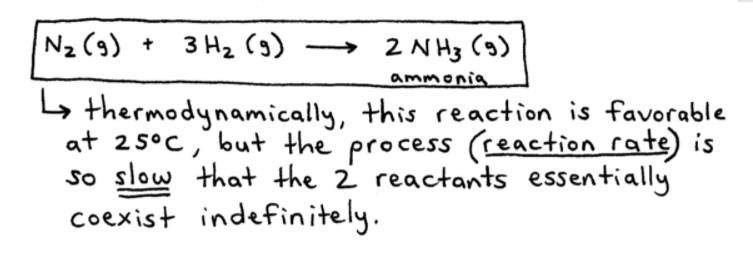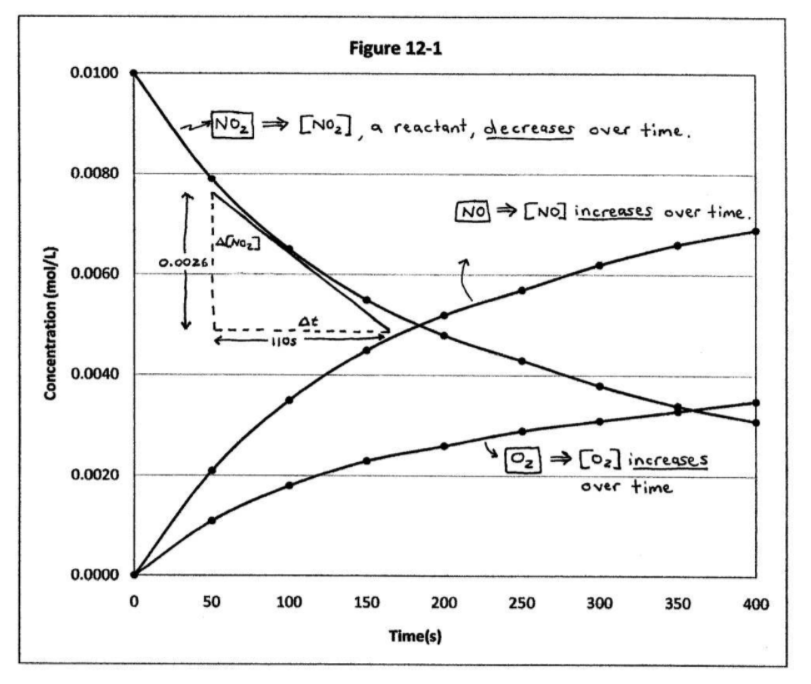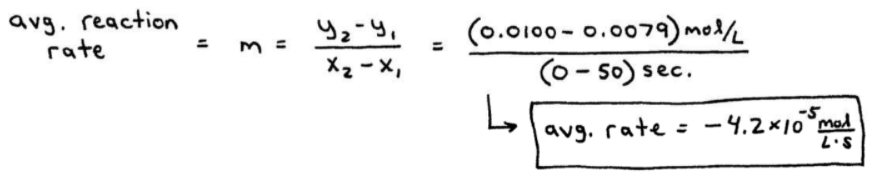# S12E1 - Chemical Kinetics and Reaction Rates

## Chemical Kinetics

Chemical Kinetics  =  the area of chemistry concerning reaction rates.

In the 1st semester of General Chemistry we learned how to write and balance chemical equations.

And using stoichiometry, we learned how to determine the amounts of reactants and/or products that were consumed and/or produced in a reaction.

➞ We learned nothing about how fast those reactions were occurring.

----------

## Why is Chemical Kinetics so Important?

The "spontaneity" of a reaction has nothing to do with how fast a reaction occurs.

While spontaneity refers to a reaction's tendency to occur, a reaction must occur at a reasonable rate to be useful.

----------

ex:  25 million tons of ammonia (NH3) are produced annually.

So how come ammonia is not produced by the following inexpensive method, where the reactants (N2 and H2) are cheap and readily available?As stated above, thermodynamically this reaction is favorable at 25°C.

But the process (reaction rate) is so slow that the 2 reactants essentially coexist indefinitely.

==========

## Kinetics and Reaction Rates

Consider the reaction presented below:

2NO2(g)  ➝  2NO(g)  +  O2(g)

Table 12.1 shows the concentrations of reactant and products over time, and the data is plotted below in Figure 12.1.Reaction Rate  =  the change in concentration of reactant(s) or products per unit time.

From above:   2NO2(g)  ➝  2NO(g)  +  O2(g)﻿We can write the reaction rate, or "rate" in terms of any of the reactants, or in terms of any of the products.

To be consistent however, it's customary to represent the reaction rate in terms of the reactant(s).

That's why you see I've boxed up and triple-starred the last equation above. Here it is again:

rate  =  - Δ[NO2] / Δt

==========

## Instantaneous Reaction Rates

An Examination of Figure 12-1 Above.

At any single point on the NO2 curve, we can calculate the instantaneous rate of the reaction.

The instantaneous rate is calculated by finding the slope (m) of the line tangent to the curve at the specific time (t).

----------

ex:  Calculate the instantaneous rate of the reaction after 100. seconds has elapsed since the reaction started.
_________

➞  we have to calculate the slope of the downward-moving NO2 curve at x = 100. s...==========

## Average Reaction Rates

ex:  Now calculate the average reaction rate at which the [NO2] reactant changes over the first 50. seconds of the reaction.
_________

➞  for the average reaction rate, we'll need to use Table 12.1 above to look at the (x,y) data points at x = 0 and x = 50. seconds.

➞  like this:----------

*NOTE:  Reaction Rate is not constant but decreases over time, as the reactant(s) concentration goes down.

In Table 12-2 below, we see that the lesser the amount of reactant remaining, the slower the reaction rate:==========

Next up in our discussion of SECTION 12 - Chemical Kinetics and Reaction Rates,